Basic matrix functions with VBA/Excel (free download)

Important update on 16. February 2019: Some links, and especially download links on this website may not work properly as I recently transferred the whole site from www.finaquant.com to software.tuncalik.com

Check my shared folder MyAnalyticalSoftware_AllDownloads for most uptodate downloads. Open-Source downloads of the C#/.NET libraries Finaquant Calcs and Finaquant Protos are also included in this shared download folder as zipped Visual Studio 2012 files. You may contact me if you need consulting for my analytical software (C#/.NET, Matlab, Python, R).This release (June 2012, V.1.1) includes about 60 basic matrix and vector functions for MS Excel users and macro (VBA, Visual Basic for Applications) programmers. All these functions are written with the native macro language (VBA) of excel.

Why would you need matrix operations in excel?

• You are a proficient excel user and programmer, and you want to do everything in excel, if possible.
• You want to implement complex matrix calculations, simulations and optimizations easily with macros (VBA) in excel.
• You want to translate the matrix functions and scripts written with mathematical applications like matlab and R into the excel VBA environment, so that you can profit from built in functions of excel like charting, reporting and data storage.

Your first VBA procedure with matrix functions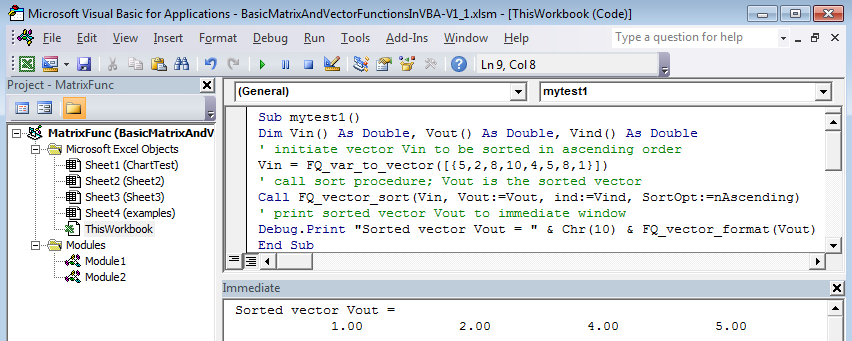Sub mytest1()
Dim Vin() As Double, Vout() As Double, Vind() As Double
Vin = FQ_var_to_vector([{5,2,8,10,4,5,8,1}])
Call FQ_vector_sort(Vin, Vout:=Vout,ind:=Vind,SortOpt:=nAscending)
Debug.Print "Sorted vector Vout = " & Chr(10) & FQ_vector_format(Vout)
End Sub

This procedure creates first a vector with 8 elements, then sorts them in ascending order, and finally prints the resultant sorted vector into the immediate window (press F5 to execute the procedure). Compare this with the corresponding matlab code:
Vin = [5,2,8,10,4,5,8,1] [Vout, Vind] = sort(Vin);
Vout

Your second VBA procedure with matrix functions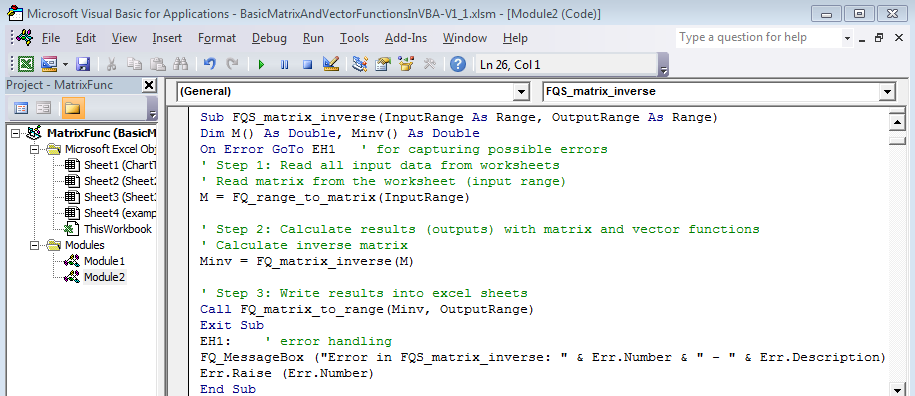This worksheet function reads the elements of a matrix from the given worksheet range (InputRange), calculates the inverse of the matrix and writes the resultant inverse matrix back into the given worksheet range (OutputRange). We can test this function with the test procedure below:
Sub TEST_FQS_matrix_inverse()
Dim r_in As Range, r_out As Range
Set r_in = ThisWorkbook.Sheets("examples").Range("A4:D7")
Set r_out = ThisWorkbook.Sheets("examples").Range("F4:G5")
Call FQS_matrix_inverse(r_in, r_out)
End SubNext steps

Already mastered matrix and vector functions in excel? Now, you are ready to advance to the next level, namely table-valued functions!

Visit: Empowering Excel with Table Valued Functions (Excel Add-in):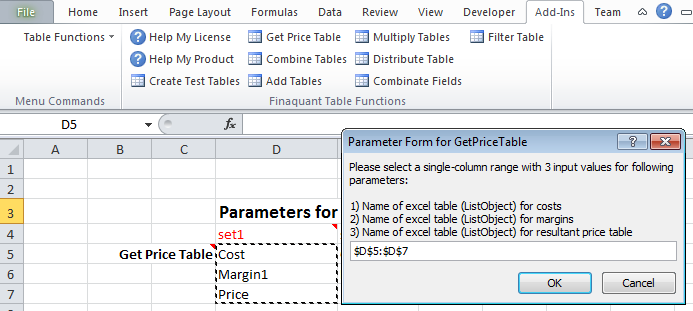Download Description FinaquantInExcel_R105.xll Excel add-in Finaquant in Excel, release R105 (digitally signed by Finaquant Analytics GmbH) IntroToFinaquantInExcel.pdf Introduction to Finaquant in Excel (add-in); a Visual Guide with table function examples and developer notes. FinaquantInExcel_R105.zip Zip package with the Visual Studio project for developing the add-in (open source)

Here is how you can contribute and get involved:

1. By bringing interesting questions and ideas to the related community forum
2. By subscribing to our news for significant release updates for matrix functions with VBA/Excel:
3. E-mail:
4. If you want, you can donate to support this project: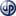Copyright secured by Digiprove © 2012 Tunc Ali Kütükcüoglu

8 Responses to Basic matrix functions with VBA/Excel (free download)

1.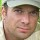tuncalik says:

Matrix determinant function is added to the new version V1.2 (July 2012). All the VBA scripts published so far for generating test data (as all possible attribute value combinations) can be found in Module3 of the downloadable excel file.

2.pmxgs0 says:

Hi,

thanks very much for these functions.
I’m not a very experienced vba user, and the functions included in the file provided by Finaquant, helped me a lot in a project where I had to use matrix operations extensively.

Keep up the good work

3.laki says:

Hi,
I am trying to download this spreadsheet to do some comparisons with some code I have written but when I click on it it looks like the download does not exist. At least this is the message I get. Any ideas why it is doing that?
Thanks a lot
Laki

•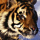admin says:

Hello Laki, apparently there was a temporary configuration error in the download manager which is then corrected. Downloads should be working by now. Thank you for your warning.

4.amiralex15 says:

thanks

5.Arto says:

How can multiply two matrices using : FQ_matrix_multiplication. I would be grateful for example as for inverse function.

6.ToParIs says:

7.loquat says: